+关注继续查看

# 《Kotlin极简教程》正式上架：

#### 非常感谢您亲爱的读者，大家请多支持！！！有任何问题，欢迎随时与我交流~

"函数式编程", 又称泛函编程, 是一种"编程范式"（programming paradigm），也就是如何编写程序的方法论。它的基础是 λ 演算（lambda calculus）。λ演算可以接受函数当作输入（参数）和输出（返回值）。

## 8.1 函数式编程概述

• 我们就从1900 年 David Hilbert 的第 10 问题（能否通过有限步骤来判定不定方程是否存在有理整数解？） 开始说起吧。

• 1920，Schönfinkel，组合子逻辑(combinatory logic)。直到 Curry Haskell 1927 在普林斯顿大学当讲师时重新发现了 Moses Schönfinkel 关于组合子逻辑的成果。Moses Schönfinkel的成果预言了很多 Curry 在做的研究，于是他就跑去哥廷根大学与熟悉Moses Schönfinkel工作的Heinrich Behmann、Paul Bernays两人一起工作，并于 1930 年以一篇组合子逻辑的论文拿到了博士学位。Curry Brooks Haskell 整个职业生涯都在研究组合子，实际开创了这个研究领域，λ演算中用单参数函数来表示多个参数函数的方法被称为 Currying (柯里化)，虽然 Curry 同学多次指出这个其实是 Schönfinkel 已经搞出来的，不过其他人都是因为他用了才知道，所以这名字就这定下来了；并且有三门编程语言以他的名字命名，分别是：Curry, Brooks, Haskell。Curry 在 1928 开始开发类型系统，他搞的是基于组合子的 polymorphic，Church 则建立了基于函数的简单类型系统。

• 1929, 哥德尔(Kurt Gödel )完备性定理。Gödel 首先证明了一个形式系统中的所有公式都可以表示为自然数，并可以从一自然数反过来得出相应的公式。这对于今天的程序员都来说，数字编码、程序即数据计算机原理最核心、最基本的常识，在那个时代却脑洞大开的创见。

• 1933，λ 演算。 Church 在 1933 年搞出来一套以纯λ演算为基础的逻辑，以期对数学进行形式化描述。 λ 演算和递归函数理论就是函数式编程的基础。

• 1936，确定性问题（decision problem，德文 Entscheidungsproblem (发音 [ɛntˈʃaɪ̯dʊŋspʁoˌbleːm]）。 Alan Turing 和 Alonzo Church，两人在同在1936年独立给出了否定答案。

1935-1936这个时间段上，我们有了三个有效计算模型：通用图灵机、通用递归函数、λ可定义。Rosser 1939 年正式确认这三个模型是等效的。

• 1953-1957，FORTRAN (FORmula TRANslating )，John Backus。1952 年 Halcombe Laning 提出了直接输入数学公式的设想，并制作了 GEORGE编译器演示该想法。受这个想法启发，1953 年 IBM 的 John Backus 团队给 IBM 704 主机研发数学公式翻译系统。第一个 FORTRAN (FORmula TRANslating 的缩写)编译器 1957.4 正式发行。FORTRAN 程序的代码行数比汇编少20倍。FORTRAN 的成功，让很多人认识到直接把代数公式输入进电脑是可行的，并开始渴望能用某种形式语言直接把自己的研究内容输入到电脑里进行运算。John Backus 在1970年代搞了 FP 语言，1977 年发表。虽然这门语言并不是最早的函数式编程语言，但他是 Functional Programming 这个词儿的创造者， 1977 年他的图灵奖演讲题为[“Can Programming Be Liberated From the von Neumann Style? A Functional Style and its Algebra of Programs”]

• 1956， LISP， John McCarthy。John McCarthy 1956年在 Dartmouth一台 IBM 704 上搞人工智能研究时，就想到要一个代数列表处理(algebraic list processing)语言。他的项目需要用某种形式语言来编写语句，以记录关于世界的信息，而他感觉列表结构这种形式挺合适，既方便编写，也方便推演。于是就创造了LISP。正因为是在 IBM 704 上开搞的，所以 LISP 的表处理函数才会有奇葩的名字： car/cdr 什么的。其实是取 IBM704 机器字的不同部分，c=content of，r=register number, a=address part, d=decrement part 。

### 8.1.1 面向对象编程（OOP）与面向函数编程（FOP）

#### 面向对象编程（OOP）

OOP 的世界提倡开发者针对具体问题建立专门的数据结构，相关的专门操作行为以“方法”的形式附加在数据结构上，自顶向下地来构建其编程世界。

OOP追求的是万事万物皆对象的理念，自然地弱化了函数。例如：函数无法作为普通数据那样来传递（OOP在函数指针上的约束），所以在OOP中有各种各样的、五花八门的设计模式。

GoF所著的《设计模式-可复用面向对象软件的基础》从面向对象设计的角度出发的，通过对封装、继承、多态、组合等技术的反复使用，提炼出一些可重复使用的面向对象设计技巧。而多态在其中又是重中之重。

#### 面向函数编程（FOP）

OOP喜欢自顶向下架构层层分解（解构），FP喜欢自底向上层层组合（复合）。 而实际上，编程的本质就是次化分解与复合的过程。通过这样的过程，创造一个美妙的逻辑之塔世界。

FP通过函数组合来构造其逻辑系统。FP倾向于把软件分解为其需要执行的行为或操作,而且通常采用自底向上的方法。函数式编程也提供了非常强大的对事物进行抽象和组合的能力。

#### 匀称的数据结构 + 匀称的算法

OOP vs FP (2).png

#### 函数与映射

f: X -> Y
p:Y -> Z
p(f) : X ->Z

fun f(x:X) : Y{}
fun p(y:Y) : Z{}
fun fp(f: (X)->Y, p: (Y)->Z) : Z {
return {x -> p(f(x))}
}


### 8.1.2 函数式编程基本特性

• 函数是"第一等公民"。
• 闭包（Closure）和高阶函数（Higher Order Function）。
• Lambda演算与函数柯里化（Currying）。
• 懒惰计算（lazy evaluation）。
• 使用递归作为控制流程的机制。
• 引用透明性。
• 没有副作用。

### 8.1.3 组合与范畴

• 对象(object).
• 态射(morphism).
• 组合(composition)操作符，

#### 组合操作符

• 结合律： f.(g.h) = (f.g).h 。

• 封闭律：如果存在态射 f, g，则必然存在 h = f.g 。

• 同一律：对结构中的每一个对象 A, 必须存在一个单位态射 Ia: A -> A， 对于单位态射，显然，对任意其它态射 f, 有 f.I = f。

• 将范畴 A 中的对象映射到范畴 B 中的对象。
• 将范畴 A 中的态射映射到范畴 B 中的态射。

image.png

#### 函数是"第一等公民"

>>> val print = fun(x:Any){println(x)}
>>> listOf(1,2,3).forEach(print)
1
2
3


#### 高阶函数（Higher order Function）

FP 语言支持高阶函数，高阶函数就是多阶映射。高阶函数用另一个函数作为其输入参数，也可以返回一个函数作为输出。

fun isOdd(x: Int) = x % 2 != 0
fun length(s: String) = s.length

fun <A, B, C> compose(f: (B) -> C, g: (A) -> B): (A) -> C {
return { x -> f(g(x)) }
}


fun main(args: Array<String>) {
val oddLength = compose(::isOdd, ::length)
val strings = listOf("a", "ab", "abc")
println(strings.filter(oddLength)) // [a, abc]
}


fun <A, B, C> compose(f: (B) -> C, g: (A) -> B): (A) -> C


fun <String, Int, Boolean> compose(f: (Int) -> Boolean, g: (String) -> Int): (String) -> Boolean


#### λ演算 （Lambda calculus 或者 λ-calculus）

𝜆 演算是函数式语言的基础。在λ-演算的基础上，发展起来的π-演算、χ-演算，成为近年来的并发程序的理论工具之一，许多经典的并发程序模型就是以π-演算为框架的。λ 演算神奇之处在于，通过最基本的函数抽象和函数应用法则，配套以适当的技巧，便能够构造出任意复杂的可计算函数。

λ演算是一套用于研究函数定义、函数应用和递归的形式系统。它由 阿隆佐·丘奇（Alonzo Church，1903~1995）和 Stephen Cole Kleene 在 20 世纪三十年代引入。当时的背景是解决函数可计算的本质性问题，初期λ演算成功的解决了在可计算理论中的判定性问题，后来根据Church–Turing thesis，证明了λ演算与图灵机是等价的。

λ 演算可以被称为最小的通用程序设计语言。它包括一条变换规则 (变量替换) 和一条函数定义方式，λ演算之通用在于，任何一个可计算函数都能用这种形式来表达和求值。

λ演算强调的是变换规则的运用，这里的变换规则本质上就是函数映射。
Lambda 表达式（Lambda Expression） 是 λ演算 的一部分。

λ演算中一切皆函数，全体λ表达式构成Λ空间，λ表达式为Λ空间到Λ空间的函数。

0 = λ f. λ x. x
1 = λ f. λ x. f x
2 = λ f. λ x. f (f x)
3 = λ f. λ x. f (f (f x))
...


0 = F x
1 = F f x
2 = F f (f x)
3 = F f (f (f x))
...



TRUE = λ x. λ y. x
FALSE = λ x. λ y. y


 I = λ x . x


>>> val I = {x:Int -> x}
>>> I(0)
0
>>> I(1)
1
>>> I(100)
100


 λ x . x + 1


( λ x . x + 1) 5


>>> val addOneLambda = {
...         x: Int ->
...         x + 1
...     }
2


>>> val addOneAnonymouse = (fun(x: Int): Int {
...         return x + 1
...     })
2


image.png

#### 函数柯里化（Currying）

f x y = x + y


map (f 2) [1, 2, 3]


Currying 用一元函数，来组合成多元函数。比如，上面的函数 f 的定义在 Scheme 里面相当于：

(define f (lambda (x) (lambda (y) (+ x y))))


fun add(x: Int, y: Int): Int {
return x + y
}



fun curryAdd(x: Int): (Int) -> Int {
return { y -> x + y }
}



val lambdaCurryAdd = {
x: Int ->
{
y: Int ->
x + y
}
}



#### 闭包（Closure）

• 要执行的代码块（由于自由变量被包含在代码块中，这些自由变量以及它们引用的对象没有被释放）
• 自由变量的作用域

Lambda表达式可以表示闭包。

#### 递归函数

1.递推:把复杂的问题的求解推到比原问题简单一些的问题的求解;
2.回归:当获得最简单的情况后,逐步返回,依次得到复杂的解。

（1）递归就是在过程或函数里面调用自身;

（2）在使用递归时,必须有一个明确的递归结束条件,称为递归出口。

fact(n) = if n=0 then 1 else n * fact(n-1)

fun factorial(n: Int): Int {
$coffee coffee>  对CoffeeScript感兴趣的读者，可以参考：http://coffee-script.org/ 但是，这个Y组合子 要是 使用 OOP 语言编程范式， 就要显得复杂许多。为了更加深刻地认识OOP 与 FP编程范式，我们使用Java 8 以及 Kotlin 的实例来说明。这里使用Java给出示例的原因，是为了给出Kotlin与Java语言上的对比，在下一章节中，我们将要学习Kotlin与Java的互操作。 首先我们使用Java的匿名内部类实现Y组合子 ： package com.easy.kotlin; /** * Created by jack on 2017/7/9. */ public class YCombinator { public static Lambda<Lambda> yCombinator(final Lambda<Lambda> f) { return new Lambda<Lambda>() { @Override public Lambda call(Object input) { final Lambda<Lambda> u = (Lambda<Lambda>)input; return u.call(u); } }.call(new Lambda<Lambda>() { @Override public Lambda call(Object input) { final Lambda<Lambda> x = (Lambda<Lambda>)input; return f.call(new Lambda<Object>() { @Override public Object call(Object input) { return x.call(x).call(input); } }); } }); } public static void main(String[] args) { Lambda<Lambda> y = yCombinator(new Lambda<Lambda>() { @Override public Lambda call(Object input) { final Lambda<Integer> fab = (Lambda<Integer>)input; return new Lambda<Integer>() { @Override public Integer call(Object input) { Integer n = Integer.parseInt(input.toString()); if (n < 2) { return Integer.valueOf(1); } else { return n * fab.call(n - 1); } } }; } }); System.out.println(y.call(10));//输出： 3628800 } interface Lambda<E> { E call(Object input); } }  这里定义了一个Lambda<E>类型， 然后通过E call(Object input)方法实现自调用，方法实现里有多处转型以及嵌套调用。逻辑比较绕，代码可读性也比较差。当然，这个问题本身也比较复杂。 我们使用Java 8的Lambda表达式来改写下匿名内部类： package com.easy.kotlin; /** * Created by jack on 2017/7/9. */ public class YCombinator2 { public static Lambda<Lambda> yCombinator2(final Lambda<Lambda> f) { return ((Lambda<Lambda>)(Object input) -> { final Lambda<Lambda> u = (Lambda<Lambda>)input; return u.call(u); }).call( ((Lambda<Lambda>)(Object input) -> { final Lambda<Lambda> v = (Lambda<Lambda>)input; return f.call((Lambda<Object>)(Object p) -> { return v.call(v).call(p); }); }) ); } public static void main(String[] args) { Lambda<Lambda> y2 = yCombinator2( (Lambda<Lambda>)(Object input) -> { Lambda<Integer> fab = (Lambda<Integer>)input; return (Lambda<Integer>)(Object p) -> { Integer n = Integer.parseInt(p.toString()); if (n < 2) { return Integer.valueOf(1); } else { return n * fab.call(n - 1); } }; }); System.out.println(y2.call(10));//输出： 3628800 } interface Lambda<E> { E call(Object input); } }  最后，我们使用Kotlin的对象表达式（顺便复习回顾一下上一章节的相关内容）实现Y组合子： package com.easy.kotlin /** * Created by jack on 2017/7/9. * * lambda f. (lambda x. (f(x x)) lambda x. (f(x x))) * * OOP YCombinator * */ object YCombinatorKt { fun yCombinator(f: Lambda<Lambda<*>>): Lambda<Lambda<*>> { return object : Lambda<Lambda<*>> { override fun call(n: Any): Lambda<*> { val u = n as Lambda<Lambda<*>> return u.call(u) } }.call(object : Lambda<Lambda<*>> { override fun call(n: Any): Lambda<*> { val x = n as Lambda<Lambda<*>> return f.call(object : Lambda<Any> { override fun call(n: Any): Any { return x.call(x).call(n)!! } }) } }) as Lambda<Lambda<*>> } @JvmStatic fun main(args: Array<String>) { val y = yCombinator(object : Lambda<Lambda<*>> { override fun call(n: Any): Lambda<*> { val fab = n as Lambda<Int> return object : Lambda<Int> { override fun call(n: Any): Int { val n = Integer.parseInt(n.toString()) if (n < 2) { return Integer.valueOf(1) } else { return n * fab.call(n - 1) } } } } }) println(y.call(10)) //输出： 3628800 } interface Lambda<E> { fun call(n: Any): E } }  #### 使用 Kotlin FP 实现 Y 组合子（Y-Combinator） 我们可以使用 Kotlin FP (Lambda, function) 写一个 Y-combinator 函数吗? Y = λf.(λx.f (x x)) (λx.f (x x))  当然，对于函数式编程也完全支持的 Kotlin 也有 FP 风格的Y 组合子实现：  /** * Created by jack on 2017/7/9. * * lambda f. (lambda x. (f(x x)) lambda x. (f(x x))) * * FP YCombinator */ // 为了方便易懂，使用 X 用做函数 (X)->Int 的别名 typealias X = (Int) -> Int // G 递归引用 G 自己 interface G : Function1<G, X> // create a fun G from lazy blocking fun G(block: (G) -> X) = object : G { // 调用函数自身 block(g) like as g(g) override fun invoke(g: G) = block(g) } typealias F = Function1<X, X> fun Y(f: F) = (fun(g: G) = g(g))(G { g -> f({ x -> g(g)(x) }) }) val fact = Y { rec -> { n -> if (n == 0) 1 else n * rec(n - 1) } } val fib = Y { f -> { n -> if (n == 1 || n == 2) 1 else f(n - 1) + f(n - 2) } } fun main(args: Array<String>) { println(fact(10)) println(fib(10)) for (i in 1..10) { println("$i!= ${fact(i)}") } for (i in 1..10) { println("fib($i) = ${fib(i)}") } }  其中，在接口 G 继承了Function1<G, X>接口： interface G : Function1<G, X>  这个Function1 接口是继承自kotlin.Function 接口： public interface Function<out R>  Function1 有一个抽象算子函数invoke ， 用来调用入参 p1 ： public interface Function1<in P1, out R> : kotlin.Function<R> { public abstract operator fun invoke(p1: P1): R }  我们定义的 G 函数，入参是block函数 (G) -> X , block函数的入参又是 G 类型，通过 invoke 函数来实现 调用自身： fun G(block: (G) -> X) = object : G { // 调用函数自身 block(g) like as g(g) override fun invoke(g: G) = block(g) }  这样，我们就可以实现一个 Y 组合子函数了： typealias F = Function1<X, X> fun Y(f: F) = (fun(g: G) = g(g))(G { g -> f({ x -> g(g)(x) }) })  我们通过 Y 组合子定义阶乘递归函数和 Fibonacci 数列函数： val fact = Y { rec -> { n -> if (n == 0) 1 else n * rec(n - 1) } } val fib = Y { f -> { n -> if (n == 1 || n == 2) 1 else f(n - 1) + f(n - 2) } }  测试代码： fun main(args: Array<String>) { val square: X = { x -> x * x } println(square(9)) println(fact(10)) println(fib(10)) for (i in 1..10) { println("$i!= ${fact(i)}") } for (i in 1..10) { println("fib($i) = \${fib(i)}")
}
}



【 Github 源码工程： https://github.com/EasyKotlin/chapter8_fp

Y combinator 给我们提供了一种方法，让我们在一个只支持first-class函数，但是没有内建递归的编程语言里完成递归。所以Y combinator给我们展示了一个语言完全可以定义递归函数，即使这个语言的定义一点也没提到递归。它给我们展示了一件美妙的事：仅仅函数式编程自己，就可以让我们做到我们从来不认为可以做到的事（而且还不止这一个例子）。

#### 引用透明性20 0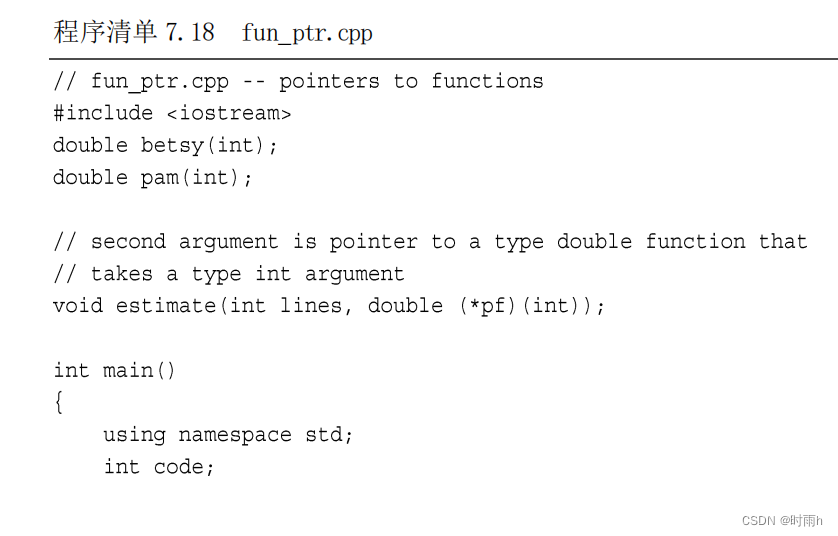C++ Primer Plus 第6版 读书笔记(7)第 7 章 函数——C++的编程模块

22 018 0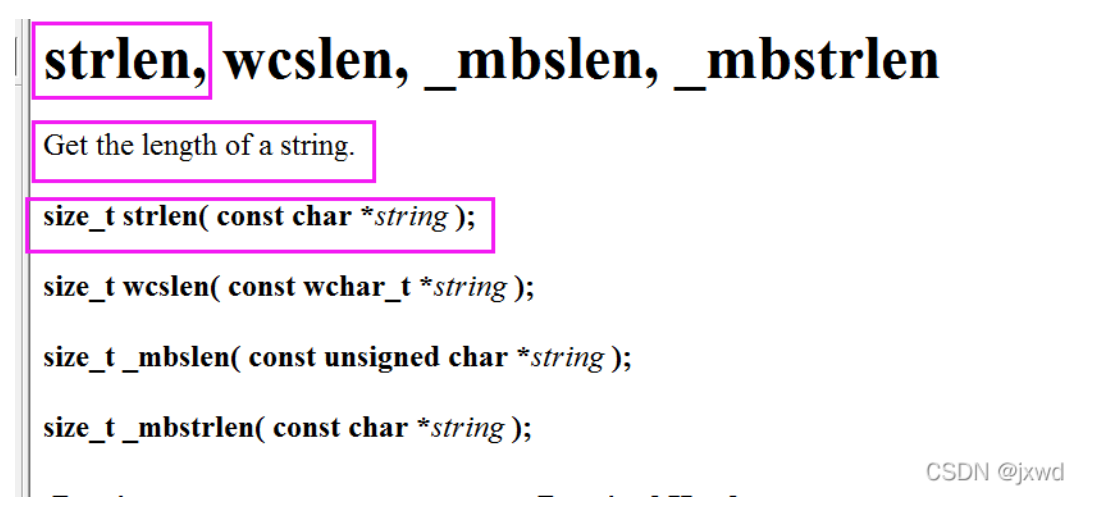0基础C语言自学教程——第十节 字符串函数

70 0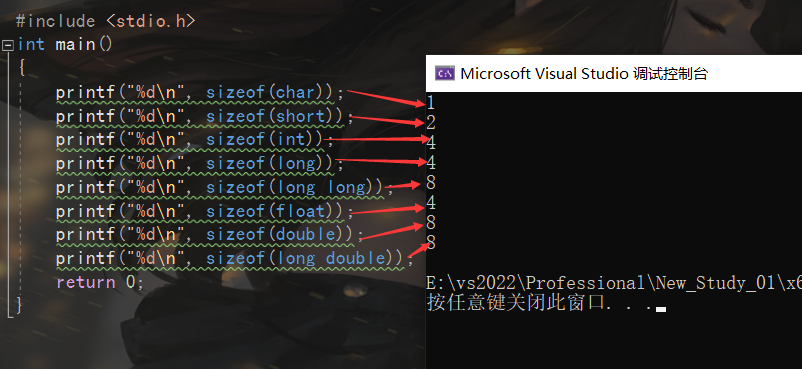66 0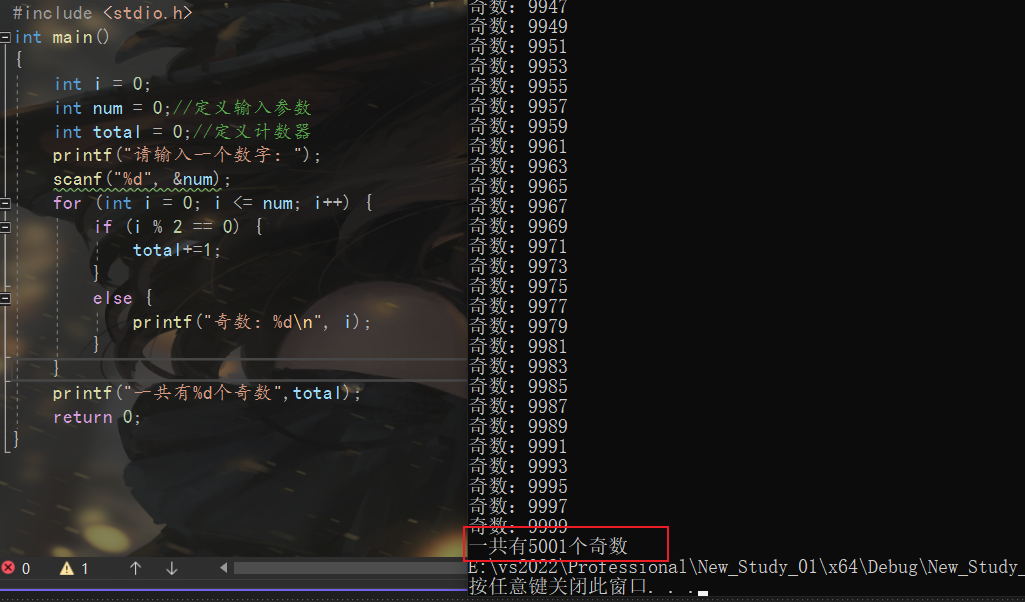56 0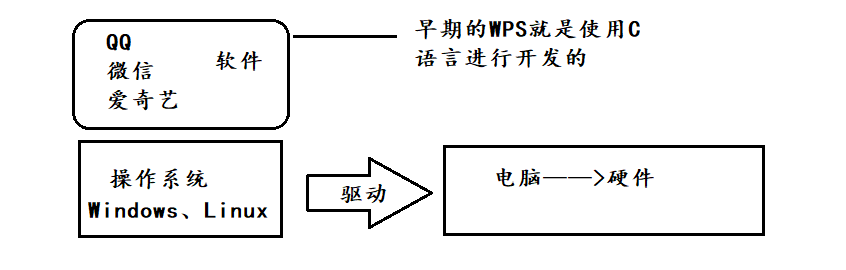128 0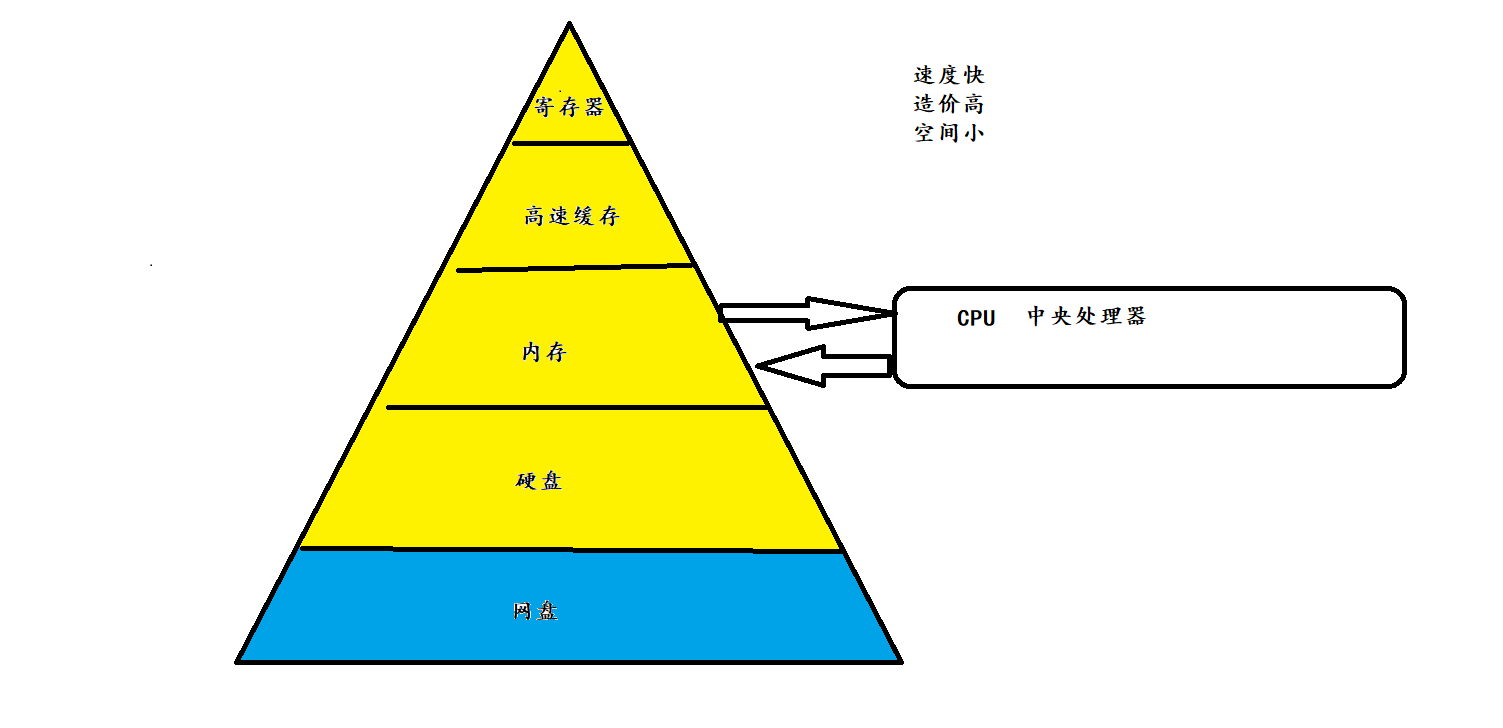50 0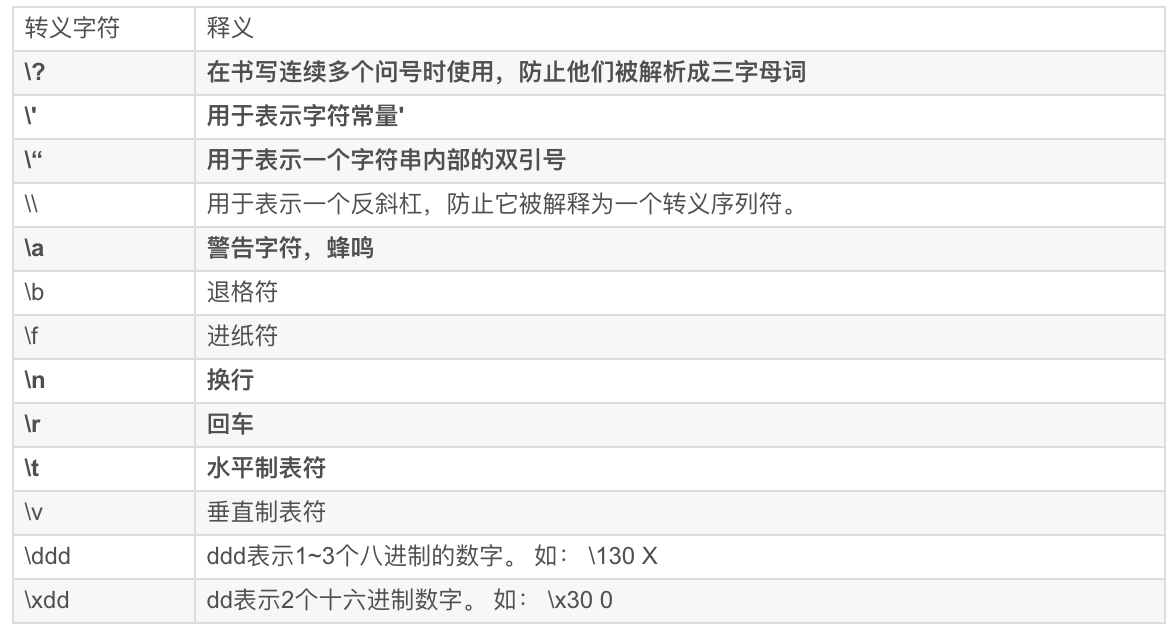145 0136 0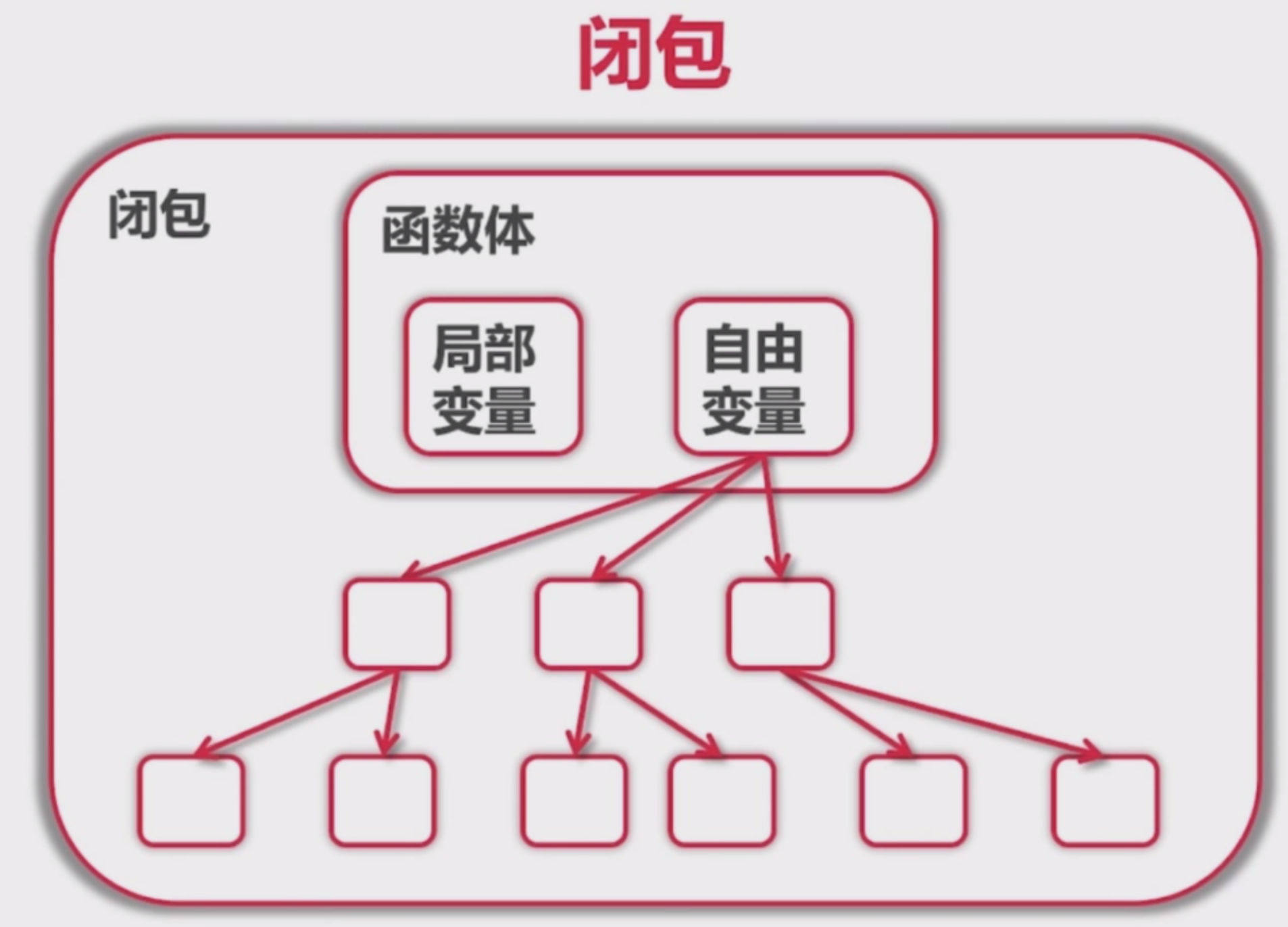38 018节视频课+编程源码揭秘Python的高阶编程之函数

2359 0C语言入门基础学习函数？来看我就告诉你！
C语言入门基础学习函数？来看我就告诉你！
1066 0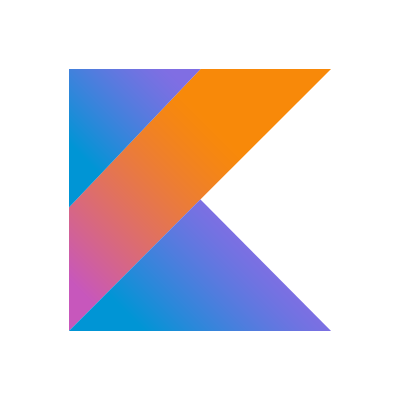kotlin极简教程 高阶函数 函数式编程章节节选
kotlin极简教程 高阶函数 函数式编程章节节选 KotlinChina编程社区 微博 《Kotlin极简教程》正式上架： 点击这里 > 去京东商城购买阅读 点击这里 > 去天猫商城购买阅读 非常感谢 if (boy) { 帅气英俊潇洒} else { 魔鬼身材天使脸蛋美丽动人女神气质} 的您。
998 0

Java面向对象编程10906148